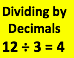Author:
Travis Rawlings
Subject:
Elementary Mathematics
Material Type:
Assessment, Game, Lesson
Level:
Upper Elementary
Tags:
Assessment, Decimals, Lesson Plan, decimals
Language:
English
Media Formats:
Text/HTML

# Dividing by Decimals## Overview

In this lesson, students will review decimal division as we prepare for our end of unit test. This will include dividing whole numbers by decimals, decimals by decimals, and decimals by whole numbers.

# Summary

In this lesson, students will review division with decimals. This will include dividing:

• Whole Numbers by Decimals
• Decimals by Decimals
• Decimals by Whole Numbers

Subject: Math

Standard: UT. Math.5.NBT.7 -Number and Operations in Base Ten

Add, subtract, multiply, and divide decimals to hundredths, using concrete models or drawings and strategies based on place value, properties of operations, and/or the relationship between addition and subtraction; relate the strategy to a written method and explain the reasoning used. In this standard, dividing decimals is limited to a whole number dividend with a decimal divisor or a decimal dividend with a whole number divisor. Compare the value of the quotient on the basis of the values of the dividend and divisor.

Time frame: 60 minutes

Format: Face-to-face

# Background for Teachers

It is helpful for the teacher to review their district's math program for dividing with decimals. For example, our district uses the iReady math program materials. So, dividing decimals is in unit 17.

This lesson is a meant to be a final review, so this material should have previously been covered.

• To teach this lesson, you will need an understanding of dividing by decimals. This includes:
• Dividing whole numbers by decimals
• Dividing decimals by decimals
• Dividing decimals by whole numbers.
• See Nearpod and YouTube videos in Section 4.

# Step 1 - Goals and OutcomesObjectives:

• Demonstrate how to divide a whole number by a decimal
• Demonstrate how to divide a decimal by a whole number
• Demonstrate how to divide a decimal by a decimal

Success Criteria:

• Students will be able to pass the end of unit assessment, which includes an example of all 3 types of decimal division.

# Step 2 - Planning InstructionStudent Background Knowledge

Students need to have previously gone through the regular class lessons on dividing with decimals. Those lessons will vary depending on your district's math program materials.

• Prior to this lesson, students will need to have an understanding of:
• The basics of division
• The basics of dividing by decimals
• Place value and how to move decimals

Strategies for Diverse Learners

Students will have the chance to work individually, as part of a team, with paper, whiteboards, and with an online Kahoot game.

Learning Interventions

Those students who are really struggling can choose or be assigned the Nearpod prior to the lesson, watch all or individual Youtube videos of Math with Mr. J, or have additional 1 on 1, or small group activities with the teacher and/or other students.

Students can choose or be assigned the following Nearpod to review prior to the lesson.

Students can also choose or be assigned some or all of the following YouTube videos from Math with Mr. J.

# Step 3 - InstructionConnection to Prior Knowledge:

1-Review what we've previously learned about division.

• Share big picture reivew of division.
• Share 3 problems on the three types of decimal devision, one at a time, on the board.
• You can pick three from your own Math curriculum
• Or use these three.
• 2.7 / 9 (Decimal by a whole number) -Answer 0.3
• 3.6 / 0.3 (Decimal by a decimal) -Answer 12
• 15 / 0.2 (Whole number by a decimal) -Answer 75
• Have students use white boards to solve.
• Have students share their thinking and answers.
• Work through the problems on the board to verify answers and model the correct procedures.

2-Pair Up.

• Have students pair up.
• Pass out the Divide Decimals Practice Sheet.
• Have students work through the 3 types of decimal division, one at a time, then share their thinking with their partner to discuss their answers.
• Review the answers as a class.

3-Kahoot Game.

• Students can stay in the current pair or divide into new pairs. This is at the teacher's discrescrion depending on how individuals and teams are working.
• Have students log-in to Kahoot to do a final 10 question review.
• Kahoot Game -Decimal Division -
• One student will run the computer and the other will use a white board to solve & verify answers.
• Students will switch places every 2 questions.

4-Assessment

• Students will take a 3 question quiz to verify their understanding of the 3 types of division using decimals. (whole number by a decimal, decimal by a decimal, decimal by a whole number.)

# Step 4 - AssessmentsStudents will have done pre-assessments with prior classwork assignments.

Formative ongoing assements will be accomplished with class:

• Discussion
• Questions
• Student reflection on individual work
• Colaborative white board work
• Group Kahoot review

Summitive end of lesson assessment will occur with a 3 question exit ticket. The end of lesson assessment will cover all 3 areas of decimal division. (Whole number by decimal, decimal by decimal, decimal by whole number)